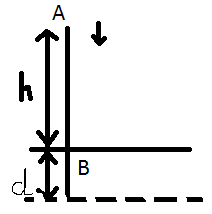# An open knife of mass m is dropped from a height h on a wooden floor. if the blade penetrates up to the depth d into the wood,average resistance offered by the wood to the knife is?????

9 years agoknife is dropped from A with u= 0 m/s

FROM A----->B

when it strikes the ground at B its velocity is:

v2 - u2 = 2as

v2- 0 = 2 (g) (h)

v= √2gh----------------------------------------(1)

after striking the ground it travels a distance ''d''

in this case u= √2gh {from (1)}

v=0

s= d

therefore, v2- u2= 2as

0 - 2gh= 2(a)(d)

-2gh/2d=a

a= -gh/d

Now, force applied by the ground is = ma= m(-gh/d) = -mgh/d.

3 years ago
On conserving mechanical energy we get velocity before penetration i.e{½mu²+mgh=½mv²}we got (v=√2gh)
Now u=√2gh
V=0
Distance=s travelled by blade during its penetration.using eqñ (2as=v²-u²)we get acceleration to be (gh/s).now drawing it free body diagram we get an eqñ(mg-N=ma)putting value of a i.e acceleration we N=mg[1+h/s].where N is normal reaction or resistive force by the wooden surface during its penetration.
2 years ago
The initial KE  + final kinetic energy+ (+ve) WD +(-ve) WD will be equal to final Work done.....
KE1+(W+)+(W-)=KEf
0+mg(h+d)-Fr(d)=0
mg(h+d)=Fr(d)
Fr=mg(1+h/d)